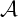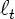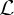### Optimal Online Prediction in Adversarial Environments (invited lecture for ALT & DS 2010)

Author: Peter L. Bartlett

Affiliation: Computer Science Division and Department of Statistics
University of California at Berkeley, Berkeley CA 94720, USA.

Abstract. In many prediction problems, including those that arise in computer security and computational finance, the process generating the data is best modelled as an adversary with whom the predictor competes. Even decision problems that are not inherently adversarial can be usefully modeled in this way, since the assumptions are sufficiently weak that effective prediction strategies for adversarial settings are very widely applicable. The first part of the talk is concerned with the regret of an optimal strategy for a general online repeated decision problem: At round t, the strategy chooses an action (possibly random) at from a set, then the world reveals a functionfrom a set, and the strategy incurs a loss. The aim of the strategy is to ensure that the regret, that is,is small. The results we present  are closely related to finite sample analyses of prediction strategies for probabilistic settings, where the data are chosen iid from an unknown probability distribution. In particular, we relate the optimal regret to a measure of complexity of the comparison class that is a generalization of the Rademacher averages that have been studied in the iid setting. Many learning problems can be cast as online convex optimization, a special case of online repeated decision problems in which the action setand the loss functionsare convex. The second part of the talk considers optimal strategies for online convex optimization [2,3]. We present the explicit minimax strategy for several games of this kind, under a variety of constraints on the convexity of the loss functions and the action set. The key factor is the convexity of the loss functions: curved loss functions make the decision problem easier. We also demonstrate a strategy that can adapt to the difficulty of the game, that is, the strength of the convexity of the loss functions, achieving almost the same regret that would be possible if the strategy had known this in advance.

References

 Abernethy, J., Agarwal, A., Bartlett, P.~L., Rakhlin, A.:
A stochastic view of optimal regret through minimax duality.
arXiv:0903.5328v1 [cs.LG] (2009)

 Abernethy, J., Bartlett, P.~L., Rakhlin, A., Tewari, A.:
Optimal strategies and minimax lower bounds for online convex games.
UC Berkeley EECS Technical Report EECS-2008-19 (2008)

 Bartlett, P.~L., Hazan, E., Rakhlin, A.: